TUCTF was a capture the flag organized by the University of Tulsa. I participated with a friend and we even managed to score first place in the high school bracket.

## WoO

Hmm. Opening the binary in radare shows some immediately suspicious calls to scanf(“%s”). It also shows that the structs containing the data about the animals are stored on the heap and it also reveals the struct layout.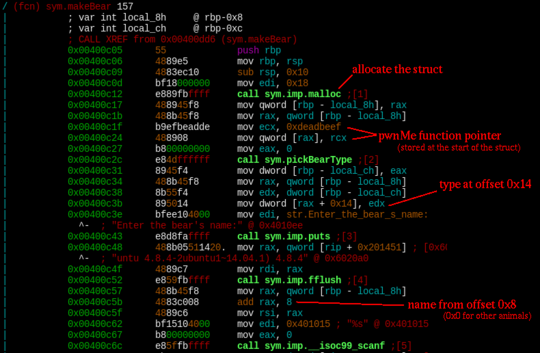There is also a secret function named pwnMe, which gets called when the number 0x1337 (4919) gets passed as a menu option and a function named l33tH4x0r (not called anywhere) which loads the flag from a file on the target system and prints it.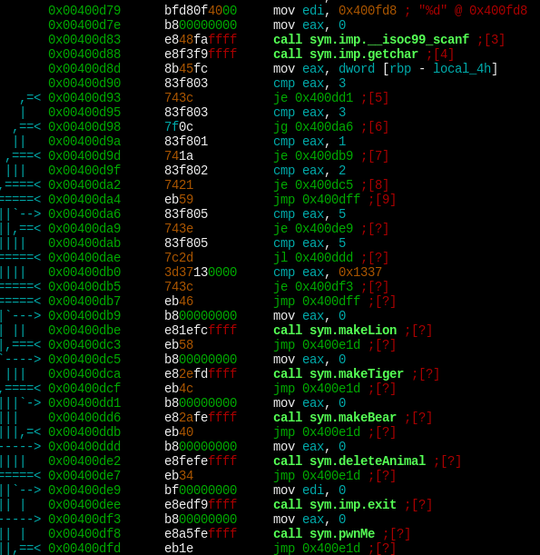Opening up the pwnMe function in gdb shows, that it loads the index of the last bear struct (which gets set in the makeBear function), checks if the type of the bear is equal to 3 and if it is, it loads a function pointer from the start of the struct and then jumps to anywhere the pointer points to.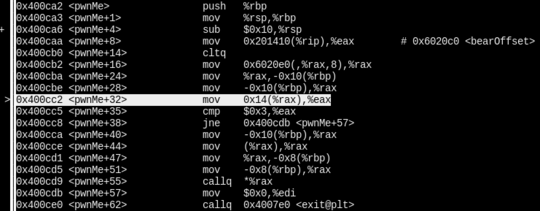The first problem is the bear type — we can choose either 1 or 2, but not 3. However, looking at the struct layout it looks like this can easily be bypassed by overflowing the bear name scanf(“%s”) as the type is placed right after it.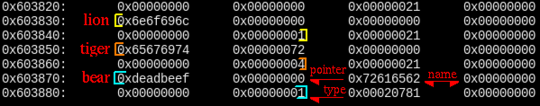Next the default 0xdeadbeef pointer needs to be overwritten, it is before the name, so overflowing the bear name won’t help.

Notice that the structs are arranged after one another on the heap - this is what the exploit relies on.

Okay, so the plan is as follows:

1. Load first two animals, does not matter which ones
2. Load a bear, overwrite its type with 3
3. Free the struct right before the bear struct
4. Overwrite the function pointer at the start of the bear struct using the scanf overflow by allocating another animal (this allocation should be put where the struct deleted in step 3. was)

The exploit then looks as follows:

## WoO2-fixed

This is a bit more complex variant of the previous challenge, as the scanf overflow is fixed, so the bear type cannot just be overwritten.

Returning to the bearOffset variable, notice that it does not get cleaned when the bear gets deleted.

Another important thing to notice is the difference in struct layout between the bear struct and the other animals — the space in the bear struct occupied by the function pointer is reused in the other animal structs for the name string.

This leads to the followint reuse-after-free scenario:

1. The bear struct gets loaded
2. The bear struct gets freed
3. Another struct takes its place, with animal type 3 and the target function pointer as its name.

## Especially Good Jmps

The challenge description also says that ASLR is enabled on the remote server.

Looking at how the name is loaded: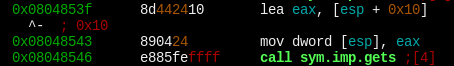Okay, so we have full control over the stack and DEP is disabled, however, as the stack location is random, we cannot just load a shellcode and then jump to it as its address is unknown.

Note that the binary is not PIE, so at least all the functions which get loaded from libc and the main application code is is accessible using constant addresses.

Let’s run a debugger and put a breakpoint at return from the main function (which is where we first are able to get control over the execution).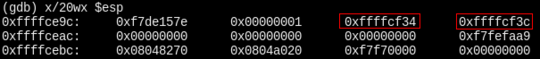Nice, so there is a stack pointer on the stack, now we need to leak it. To do that, a fragment of the “Hello %s, %d is an odd number!” can be used (remember, the binary is not position independent, so this will stay on a constant address) and an address of the printf function from the PLT.

In the first round of the exploit, the stack will be arranged as follows:

1. Pointer to printf in the PLT
2. main address (as we need to run main one more time after leaking the pointer)
3. Pointer to “%d is an odd number”
4. Some stack pointer

This will cause the server to output the stack pointer, the second round is then easy — just load a pointer to the shellcode and the shellcode itself.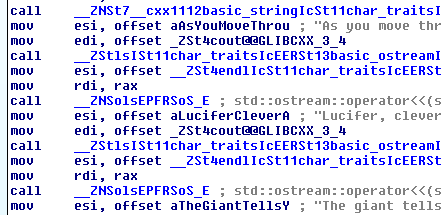Opening up the file in IDA immediately shows that it was written in C++. This is as good as an obfuscation, especially because this is the first time I am reversing anything written in it.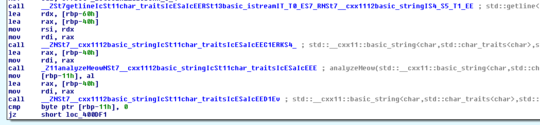The task is to figure out what input to the analyzeMeow function will make it return true. The important parts of the function are here: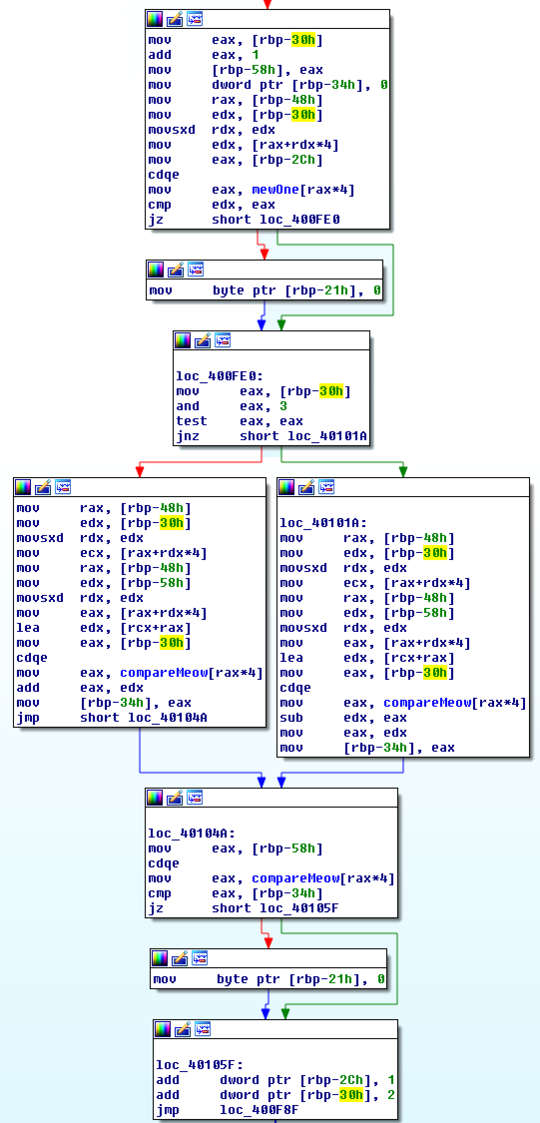The first part is easy to puzzle out — compare every character of the input at an odd index to the characters stored in mewOne. This leads to:

After staring at the second part for a bit, I decided to take a more straigtforward approach:

The point at which the comparison fails is at the mov [rbp-25h], 0 line. The current character index is stored at [rbp-0x2c]. This means that the flag can easily be guessed character-by-character by setting a breakpoint to trigger when the comparison fails and checking whether the counter incremented beyond the currently guessed character.

Altough this could very likely be easily implemented by scripting gdb directly, there was not enough of time for me to learn that, so I just took a semi-automatic approach:

And in another window:

## Secure Auth

Okay, here we have an RSA signing oracle (it obviously won’t sign the requested message directly). However, this oracle is not using proper padding, which has a lot of problems.

First, the public key needs to be recovered, this can be done using a pair of two signatures, $$(m_1, s_1),\ (m_2, s_2)$$:

$m_i = s^e_i \ \bmod \ n \\ s^e_i = k_in + m_i \\ \gcd(s_1^e - m_1, s_2^e - m_2) = \gcd(k_1, k_2) n$

This also means guessing the public exponent, which turns out to be the commonly used $$65537$$. Altough $$\gcd(k_1, k_2)$$ is not guaranteed to be $$1$$ it is easy to just pick new message pairs until it is (the public key then can be verified by getting the server to sign it, as $$N^d \bmod N = 0$$).

Now onto the signature forgery. We first need to pick a constant $$k$$ such as that the message ($$m_b$$) is evenly divisible by it. Signatures for $$k m_b$$ and $$k$$ can then be used to create a valid signature for $$m_b$$.

\begin{align} s_k &= k^d \bmod N \\ s_m &= (km_b)^d \bmod N \\ &\Rightarrow \\ s_b &= s_m s_k^{-1} \bmod N \end{align}

## Hash n Bake

So, here we are given $$hash(xor(k, m_1))$$ and $$m_2$$ and need to compute $$hash(xor(k, m_2))$$. As it looks like, we need to inverse the hash function and then xor PLAIN_1 with the result to get KEY.

Looking at the hash function, there is an important observation to make:

The last bit of the result is flipped (compared to CONST) if and only if the message got XORed in the final round.

After un-xoring it, the same applies to the previous bit and so on, so the inverse hash function looks as follows:

Another important observation is, that the first bit of CONST2 is 1 – this means that the message can safely be initialized to zeroes, as that is what it ends up being after the hash function runs forward.

To make this a bit more clear, let’s take a look at some intermediate values:

(exclamation marks show when the result has been xored)

## Update: Prizes

The prizes have finally arrived!